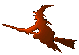Hex  1.0 Hydrogen-electron collision solver
CompactIntegrand< Functor, FType > Class Template Reference

Compactification multiplied by its jacobian. More...

#include <compact.h>

## Public Member Functions

CompactIntegrand (Functor f, double a=0., double b=special::constant::Inf, bool limit=true, double L=1.0)

~CompactIntegrand ()

FType operator() (double t) const

double scale (double x) const
Scale value from the original interval [a,b] into compactified interval [-1,1]. More...

double unscale (double t) const
Unscale value from the compactified interval [-1,1] into the original interval [a,b]. More...

## Detailed Description

### template<class Functor, typename FType> class CompactIntegrand< Functor, FType >

A wrapper around the compactification classes which returns function value multiplied by the Jacobian of the compactification transformation. It is meant for the use in integration, so that one can just call its operator() interface instead of operator()*jacobian

## Constructor & Destructor Documentation

template<class Functor, typename FType>
 CompactIntegrand< Functor, FType >::CompactIntegrand ( Functor f, double a = 0., double b = special::constant::Inf, bool limit = true, double L = 1.0 )
inline

Constructor of the class The parameters "a" and "b" specify original definition interval [a,b]. It must be a < b.

template<class Functor, typename FType>
 CompactIntegrand< Functor, FType >::~CompactIntegrand ( )
inline

## Member Function Documentation

template<class Functor, typename FType>
 FType CompactIntegrand< Functor, FType >::operator() ( double t ) const
inline

Get the function multiplied by the Jacobian of the transform for the purpose of integrating the original function.

Note
Whenever the function value is zero, the Jacobian is not evaluated and a clean zero is returned.
template<class Functor, typename FType>
 double CompactIntegrand< Functor, FType >::scale ( double x ) const
inline
template<class Functor, typename FType>
 double CompactIntegrand< Functor, FType >::unscale ( double t ) const
inline

The documentation for this class was generated from the following file: